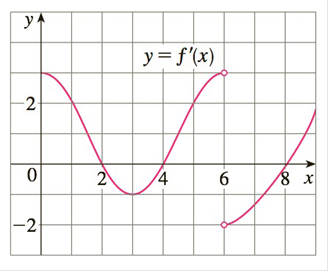Chapter 3.3, Problem 31E

Chapter
Section
Textbook Problem

# 31-32 The graph of the derivative f′ of a continuous function f is shown.(a) On what intervals is f increasing? Decreasing?(b) At what values of x does f have a local maximum? Local minimum?(c) On what intervals is f concave upward? Concave downward?(d) State the x-coordinate(s) of the point(s) of inflection.(e) Assuming that f ( 0 ) = 0 , sketch a graph of f.To determine

(a)

To find:

The interval on which f is increasing and decreasing

Explanation

1) Concept:

Increasing or Decreasing test:

If f'x>0 then f is increasing

If f'x<0 then f is decreasing

2) Calculation:

The given graphis a graph of f'(x)

Therefore, by using Increasing or decreasing test if f'x>0 then f is increasing and if

f'x<0 then f is decreasing

Now, from the given graph we find f'x>0 on the interval 0, 24, 68,

To determine

(b)

To find:

The values of x at which f has local maximum and local minimum

To determine

(c)

To find:

On what interval f is concave upward and concave downward

To determine

(d)

To state:

The x-coordinate(s) of the point(s) of inflection

To determine

(e)

To sketch:

The graph of f assuming that f0=0

### Still sussing out bartleby?

Check out a sample textbook solution.

See a sample solution

#### The Solution to Your Study Problems

Bartleby provides explanations to thousands of textbook problems written by our experts, many with advanced degrees!

Get Started

#### Find more solutions based on key concepts